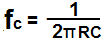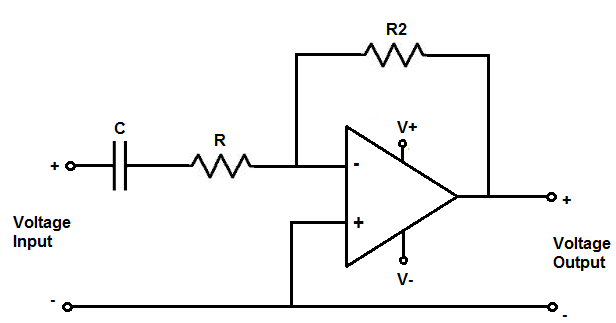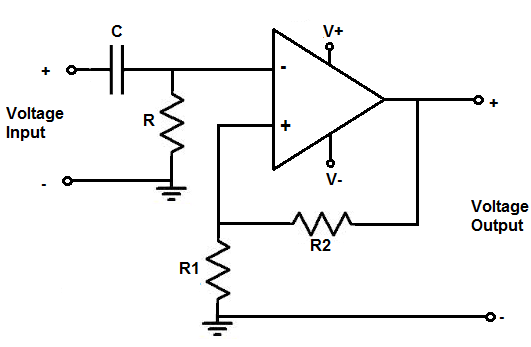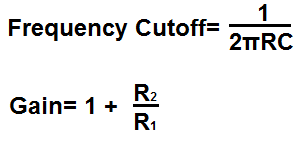﻿ High Pass Filter Calculator ﻿# High Pass Filter Calculator### RC High Pass Filter (Passive)### RC High Pass Filter Calculator

This passive RC high pass filter calculator calculates the cutoff frequency point of the high pass filter, based on the values of the resistor, R, and the capacitor, C, of the circuit, according to the formula fc= 1/(2πRC).

To use this calculator, all a user must do is enter any 2 values in the field, and the calculator will compute the value of third field. This calculator allows a user to select the magnitude of the units of the capacitor, including picofarads (pF), nanofarads (nF), microfarads (µF), and farads (F), along with the units for the resistance and frequency. After the user has entered in 2 values, he or she clicks the 'Calculate' button, and the result is automatically computed. The resultant value of the cutoff frequency calculated is in unit hertz (Hz) for frequency, unit farads for capacitance, and unit ohms for resistance.

An RC high pass filter is a filter circuit, composed of a resistor and a capacitor, which passes high-frequency signals and blocks low frequency signals. When a capacitor is placed in series with the power source of the circuit and a resistor is placed in parallel to that same power source, as shown in the diagram circuit above, this type of circuit forms a high pass filter. It forms a high pass filter because of the reactive properties of a capacitor. A capacitor is a reactive device. This means that the resistance that it offers to a signal changes depending on the frequency of the signal. Capacitors are reactive devices that offer very high resistance, or impedance, to low frequency signals. Conversely, they offer lower resistance as the frequency of the signal increases. Being that a capacitor offers very high resistance to low frequency signals, when placed in series with a power source, they block low-frequency signals from entering and going on to output. However, high-frequency signals are able to go through unimpeded, as capacitors offer very low resistance to them. This is how an RC high filter circuit works.

When we calculate the cutoff frequency of a high pass filter, which is what this calculator does, we're calculating the point in the frequency response of the filter, where the gain of the signal reaches half of the power of the full strength signal it will later be. This is the point when the gain is 3dB off from being at its full strength. As the frequency increases, the gain will increase until it reaches its full strength. This can be seen from the diagram above. The cutoff frequency of a high pass filter is crucial because it shows the point where the gain is 50% of its full (peak) power.

As you can see in the above diagram, the high pass filter begins at 0 at the lowest end of the frequency spectrum. This is because high-pass filters can produce barely any gain at all for low frequency signals, being that low-frequency signals are filtered out. As the frequency increases, so does the gain. At the cutoff frequency, the signal is just 3dB from its full gain. As the frequency increases more, the gain reaches full strength.

### RL High Pass Filter (Passive)### RL High Pass Filter Calculator

This passive RL high pass filter calculator calculates the cutoff frequency point of the high pass filter, based on the values of the resistor, R, and inductor, L, of the circuit, according to the formula fc= R/(2πL).

To use this calculator, all a user must do is enter in any 2 values, and the calculator will compute the third field. This calculator allows a user to select the magnitude of the units of the inductor, including picohenry (pH), nanohenry (nH), microhenry (µH), and henry (H). After the 2 values are entered in, the user clicks the 'Calculate' button, and the result is automatically computed. The resultant value of the cutoff frequency calculated is in unit hertz for frequency, unit henries for inductance, and unit ohms for resistance.

An RL high pass filter is a filter circuit, composed of a resistor and an inductor, which passes high-frequency signals and blocks low frequency signals. When an resistor is placed in series with the power source of the circuit and an inductor is placed in parallel to that same power source, as shown in the diagram circuit above, this type of circuit forms a high pass filter. It forms a high pass filter because of the reactive properties of an inductor. An inductor is a reactive device. This means that the resistance that it offers to a signal changes depending on the frequency of the signal. Inductors are reactive devices that offer very high resistance, or impedance, to high frequency signals. Conversely, they offer higher resistance as the frequency of the signal decreases. Thus, an inductor offers very high impedance to a very high frequency signal. Because they offer high impedance to high frequency signals, high frequency signals do not go through them, as they represent a high-impedance path. Remember that current always takes the path of least resistance. So high-frequency signals normally do not take the inductor path; instead, they go through to the output, which is why this filter is called a high pass filter. Low frequency signals, on the other hand, do go through the inductor, as inductors offer low-frequency signals low impedance. So low-frequency take the inductor path and do not go on through the output path.

When we calculate the cutoff frequency of a high pass filter, which is what this calculator does, we're calculating the point in the frequency response of the filter, where the gain of the signal is half of the power of the full (peak) strength signal. This is the point when the gain is 3dB off from being at its full strength. As the frequency increases, the gain will increase until it reaches its full strength. This can be seen from the diagram above. The cutoff frequency of a high pass filter is crucial because it shows the point where the gain is 50% of its full power.

As you can see in the above diagram, the high pass filter begins at 0 at the lowest end of the frequency spectrum. This is because high-pass filters can produce barely any gain at all for low frequency signals, being low-frequency signals are filtered out. As the frequency increases, so does the gain. At the cutoff frequency, the signal is just 3dB from its full gain. As the frequency increases more, the gain reaches full strength.

### Inverting Op Amp High Pass Filter (Active)Enter the Cutoff Frequency Desired Hz (hertz) KHz (kilohertz) MHz (megahertz) Enter the Gain Desired

This calculator is for an active inverting op amp high pass filter.

This op amp high pass filter produces an amplified inverting signal at the output. This means that the output signal is 180 degrees out of phase with the input signal.

Resistor R and capacitor C form the cutoff frequency point.

Any frequencies above this frequency point will pass through to output amplified. Frequencies below this point will be greatly attenuated.

The formula for calculating the cutoff frequency is, frequency= 1/2πR2C

The gain of the circuit is determined by resistor R2 and resistor R, according to the formula, gain (AV)= -R2/R.

The negative sign means the output is the inverted signal of the input. This means whenver the input signal is ON, the output signal is OFF. They're exactly 180 degrees out of phase.

For this calculator, a user just has to enter the cutoff frequency and the gain desired. The calculator will then compute the resistor R, capacitor C, and resistor R2.

An important rule to keep in mind for this calculator if you are using a specific op amp is that you have to consider the op amp's specification when building the circuit.

The 2 specifications of the op amp that must be considered are the maximum DC voltage that can be supplied to the power rails of the op amp and the slew rate of the op amp.

First, you must know the maximum DC voltage that the op ap can handle at the power pins. This will allow us to know the maximum voltage that the AC voltage can swing from peak to peak. If, for example, the maximum DC voltage that the op amp can handle is ±18V, this means that the maximum AC voltage that the op amp can output is 36 volts peak to peak, or 18V peak. The AC voltage can only go as high as the DC rail. So if we feed +18V into V+ and -18V into V-, the AC voltage can swing as high as +18V and as low as -18V, which is 36 volts peak to peak. So the maximum DC voltage shows the maximum AC voltage it can show. If the AC voltage is larger than the DC rail, there will be clipping and distortion in the output signal.

The other factor is the op amp's slew rate. The op amp's slew rate is how fast the op amp can output voltage per a given unit of time. If the voltage is too large for a given frequency, the op amp may not be able to keep up and it will produce distorted. The slew rate of the op amp allows you to calculate the amount of voltage the op amp can output for a given frequency.

Slew rates range widely from op amp to op amp. The LM741 op amp has a slew rate of 0.5V/μS. High-speed op amps can have slew rates up to 6000V/μS.

How you can calculate if the op amp can handle a certain voltage at a certain frequency is determined by the formula, slew rate= 2πfV. The best way to do it is to convert the slew rate from volts per microsecond to volts per second. You do this by dividing the voltage by 0.000001 (a microsecond). Using the LM741's slew rate of 0.5V/μS, this would be 500,000V/s. We then plug this into the slew rate formula, slew rate= 2πfV= 500,000= 2(3.14)f(10V)= 7961Hz. So the op amp can output 10V at a maximum frequency of 7961Hz. Any frequency above this at 10V and the op amp won't be able to keep up with the output voltage. The frequency would be too fast for that voltage. Therefore, the op amp wouldn't be able to output that amplitude of voltage at that speed (frequency). So the slew rate must definitely be considered when creating this circuit.

If you are using relatively high frequencies, you will need a high-speed op amp.

### Noninverting Op Amp High Pass Filter (Active)Enter the Cutoff Frequency Desired Hz (hertz) KHz (kilohertz) MHz (megahertz) Enter the Gain Desired

This calculator is for an active noninverting op amp high pass filter.

This op amp high pass filter produces a noninverting signal at the output. This means that the output signal is exactly in phase with the input signal.

Resistor R and capacitor C set the cutoff frequency point.

Frequencies above this cutoff frequency get passed to output. Frequencies below this cutoff frequency point are greatly attenuated.

The formula for calculating the cutoff frequency is, frequency= 1/2πRC

The resistor R2 and resistor R1 determine the gain of the circuit. The gain of the circuit is determined by the formula, gain (AV)= 1+ R2/R1.

For this calculator, a user just has to enter the cutoff frequency and the gain desired. The calculator will then compute the resistor R, capacitor C, resistor R2, and resistor R1.

Just as with the other op amp filter circuit, the specifications of this op amp must be considered.

Related Resources

﻿